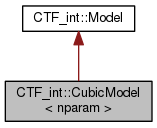Cyclops Tensor Framework parallel arithmetic on multidimensional arrays
CTF_int::CubicModel< nparam > Class Template Reference

Cubic performance models, which given measurements, provides new model guess. More...

`#include <model.h>`

Inheritance diagram for CTF_int::CubicModel< nparam >:[legend]
Collaboration diagram for CTF_int::CubicModel< nparam >:[legend]

## Public Member Functions

CubicModel (double const *init_guess, char const *name, int hist_size=8192)
constructor More...

~CubicModel ()

void update (MPI_Comm cm)
updates model based on observarions More...

void observe (double const *time_param)
records observation consisting of execution time and nparam paramter values More...

bool should_observe (double const *time_param)
decides whether the current instance should be observed More...

double est_time (double const *param)
estimates model time based on observarions More...

void print ()
prints current parameter estimates More...

void print_uo ()
prints time estimate errors More...

double * get_coeff ()
return the turned model coefficients More...

void load_coeff (std::string file_name)
load model coefficients from file More...

void write_coeff (std::string file_name)
write model coefficients to file More...

void dump_data (std::string path)
write model coefficients to file More...

## Detailed Description

### template<int nparam> class CTF_int::CubicModel< nparam >

Cubic performance models, which given measurements, provides new model guess.

Definition at line 144 of file model.h.

## Constructor & Destructor Documentation

template<int nparam>
 CTF_int::CubicModel< nparam >::CubicModel ( double const * init_guess, char const * name, int hist_size = `8192` )

constructor

Parameters
 [in] init_guess array of size nparam consisting of initial model parameter guesses [in] name identifier [in] hist_size number of times to keep in history

Definition at line 763 of file model.cxx.

template<int nparam>
 CTF_int::CubicModel< nparam >::~CubicModel ( )

Definition at line 768 of file model.cxx.

## Member Function Documentation

template<int nparam>
 void CTF_int::CubicModel< nparam >::dump_data ( std::string path )
virtual

write model coefficients to file

Parameters
 [in] path the path that we wish to dump all files to

Reimplemented from CTF_int::Model.

Definition at line 821 of file model.cxx.

References CTF_int::LinModel< nparam >::dump_data().

template<int nparam>
 double CTF_int::CubicModel< nparam >::est_time ( double const * param )

estimates model time based on observarions

Parameters
 [in] param array of size nparam of form [val_1,val_2,...,val_nparam]
Returns
estimated time

Definition at line 789 of file model.cxx.

References CTF_int::LinModel< nparam >::est_time().

template<int nparam>
 double * CTF_int::CubicModel< nparam >::get_coeff ( )

return the turned model coefficients

Definition at line 806 of file model.cxx.

References CTF_int::LinModel< nparam >::get_coeff().

template<int nparam>
 void CTF_int::CubicModel< nparam >::load_coeff ( std::string file_name )
virtual

load model coefficients from file

Parameters
 [in] file_name the file we wish to load model coefficients from

Reimplemented from CTF_int::Model.

Definition at line 811 of file model.cxx.

References CTF_int::LinModel< nparam >::load_coeff().

template<int nparam>
 void CTF_int::CubicModel< nparam >::observe ( double const * time_param )

records observation consisting of execution time and nparam paramter values

Parameters
 [in] time_param array of size nparam+1 of form [exe_sec,val_1,val_2,...,val_nparam]

Definition at line 776 of file model.cxx.

References CTF_int::LinModel< nparam >::observe().

template<int nparam>
 void CTF_int::CubicModel< nparam >::print ( )
virtual

prints current parameter estimates

Reimplemented from CTF_int::Model.

Definition at line 796 of file model.cxx.

References CTF_int::LinModel< nparam >::print().

template<int nparam>
 void CTF_int::CubicModel< nparam >::print_uo ( )
virtual

prints time estimate errors

Reimplemented from CTF_int::Model.

Definition at line 801 of file model.cxx.

References CTF_int::LinModel< nparam >::print_uo().

template<int nparam>
 bool CTF_int::CubicModel< nparam >::should_observe ( double const * time_param )

decides whether the current instance should be observed

Parameters
 [in] time_param array of size nparam+1 of form [exe_sec,val_1,val_2,...,val_nparam]

Definition at line 784 of file model.cxx.

template<int nparam>
 void CTF_int::CubicModel< nparam >::update ( MPI_Comm cm )
virtual

updates model based on observarions

Parameters
 [in] cm communicator across which we should synchronize model (collect observations)

Reimplemented from CTF_int::Model.

Definition at line 771 of file model.cxx.

References CTF_int::LinModel< nparam >::update().

template<int nparam>
 void CTF_int::CubicModel< nparam >::write_coeff ( std::string file_name )
virtual

write model coefficients to file

Parameters
 [in] file_name the file we wish to write model coefficients to

Reimplemented from CTF_int::Model.

Definition at line 816 of file model.cxx.

References CTF_int::LinModel< nparam >::write_coeff().

The documentation for this class was generated from the following files: